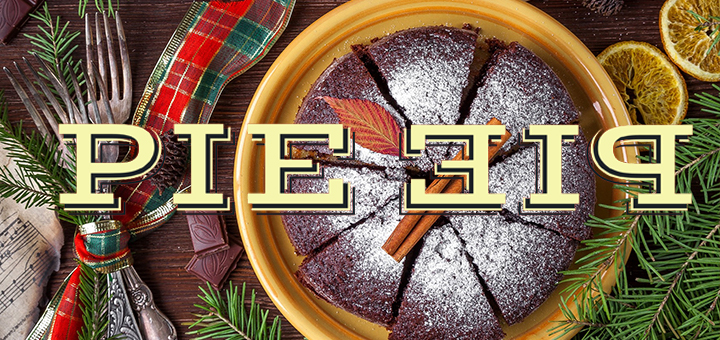# A One-Line Proof that there are Infinitely Many Prime Numbers## Problem 446

Prove that there are infinitely many prime numbers in ONE-LINE.Add to solve later

## Background

There are several proofs of the fact that there are infinitely many prime numbers.
Proofs by Euclid and Euler are very popular.

In this post, I would like to introduce an elegant one-line proof published by Sam Northshield in 2015.

Because the published paper really contains only one line, it is hard to cite only a part of it.
So I decided to cite all of his proof word for word.

## Proof by Sam Northshield (2015).

If the set of primes is finite, then
$0 < \prod_p \sin \left(\, \frac{\pi}{p} \,\right)=\prod_p \sin\left(\, \frac{\pi\cdot (1+2\prod_{p'}p')}{p} \,\right)=0 \tag{*}.$

## More details in this one-line proof.

The above proof was given by Sam Northshield in 2015.
Let us give more details hidden in this one line proof.

Suppose that there are only finitely many prime numbers $p_1, p_2, \dots, p_n$.
Since prime numbers must be greater than $1$, we have
$\sin\left(\, \frac{\pi}{p_i} \,\right) > 0$ for any $i=1, \dots, n$.
Thus, the product
$\prod_{i=1}^n\sin\left(\, \frac{\pi}{p_i} \,\right)$ is still positive since the product of finitely many positive numbers is positive.
This explains the first inequality in (*).

Recall the following basic property of the sine function.
For any integer $m$, we have
$\sin(\theta+2\pi m)=\sin(\theta)$ for any $\theta \in \R$.

We have
\begin{align*}
\frac{\pi\cdot (1+2\prod_{j=1}^n p_j)}{p_i} &=\frac{\pi}{p_i}+\frac{2\pi \prod_{j=1}^n p_j}{p_i}.
\end{align*}
Note that since $\prod_{j=1}^n p_j$ is the product of all $p_1, \dots, p_n$, one factor is $p_i$.
Hence
$m_i:=\frac{\prod_{j=1}^n p_j}{p_i}$ is just an integer, not a fraction.
It follows from this observation that we have for each $i=1, \dots, n$
\begin{align*}
\sin\left(\, \frac{\pi\cdot (1+2\prod_{j=1}^n p_j)}{p_i} \,\right)&=\sin\left(\, \frac{\pi}{p_i}+2\pi m_i \,\right)\\
&=\sin\left(\, \frac{\pi}{p_i} \,\right)
\end{align*}
by the property of the sine function mentioned above.

Taking the product of these over all $i=1, \dots, n$, we obtain
\begin{align*}
\prod_{i=1}^n \sin \left(\, \frac{\pi}{p_i} \,\right)=\prod_{i=1}^n \sin\left(\, \frac{\pi\cdot (1+2\prod_{j=1}^n p_j)}{p_i} \,\right).
\end{align*}
This is the first equality in (*).

To see the last equality in (*), we consider the number
$1+2\prod_{j=1}^n p_j$ in the numerator.

If this is a prime number, then it must be one of $p_1, \dots, p_n$.
If it is not a prime number, then it is divisible by some prime number $p_1, \dots, p_n$.
In either case, there is a prime number $p_{i_0}$ among $p_1, \dots, p_n$ such that
$\frac{1+2\prod_{j=1}^n p_j}{p_{i_0}}$ is an integer.
Therefore, we have
$\sin\left(\, \frac{\pi\cdot (1+2\prod_{j=1}^n p_j)}{p_{i_0}} \,\right)=0.$

Since one of the factors is zero, the product
$\prod_{i=1}^n \sin\left(\, \frac{\pi\cdot (1+2\prod_{j=1}^n p_j)}{p_i} \,\right)$ is also zero.
This proves the last equality in (*).
The inequality obtained in (*) is clearly a contradiction.
Hence there must be infinitely many prime numbers.
This completes the proof.

## Comment.

The one-line proof of Northshield is very clever and elegant.
But to explain the proof to high-school students, I feel that I need to probably decipher the proof and give more explanations as I did in this post.

Once you understand the details, the one-line proof is a very convenient and beautiful way to hide the details.

## Reference

The one-line proof was published in the paper

Northshield, Sam. A one-line proof of the infinitude of primes.
Amer. Math. Monthly 122 (2015), no. 5, 466.Add to solve later

### 1 Response

1. 06/08/2017

[…] A One-Line Proof that there are Infinitely Many Prime Numbers. […]

This site uses Akismet to reduce spam. Learn how your comment data is processed.

###### More in General##### Beautiful Formulas for pi=3.14…

The number $\pi$ is defined a s the ratio of a circle's circumference $C$ to its diameter $d$: $\pi=\frac{C}{d}.$ $\pi$...

Close